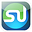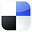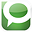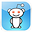# Regression AnalysisDone in ExcelHow To Read the Output

There is a lot more to the Excel Regression output than just the regression equation. If you know how to quickly read the output of a Regression done in, you’ll know right away the most important points of a regression: if the overall regression was a good, whether this output could have occurred by chance, whether or not all of the independent input variables were good predictors, and whether residuals show a pattern (which means there’s a problem).

Excel Regression Output With Color-Coding Added

Click On Image To See Enlarged View

This video will illustrate exactly how to quickly and easily understand the output of Regression performed in Excel:

Step-By-Step Video About How To Quickly Read and Understand the Output of Excel Regression

The 4 Most Important Parts of Regression Output
1) Overall Regression Equation’s Accuracy
(R Square and Adjusted R Square)

2) Probability That This Output Was Not By Chance
(ANOVA – Significance of F)

3) Individual Regression Coefficient and Y-Intercept Accuracy

4) Visual Analysis of Residuals

Some parts of the Excel Regression output are much more important than others. The goal here is for you to be able to glance at the Excel Regression output and immediately understand it, so we will focus our attention only on the four most important parts of the Excel regression output.

1) Overall Regression’s Accuracy

Click On Image To See Enlarged View

R Square– This is the most important number of the output. R Square tells how well the regression line approximates the real data. This number tells you how much of the output variable’s variance is explained by the input variables’ variance. Ideally we would like to see this at least 0.6 (60%) or 0.7 (70%).

Adjusted R Square – This is quoted most often when explaining the accuracy of the regression equation. Adjusted R Square is more conservative the R Square because it is always less than R Square. Another reason that Adjusted R Square is quoted more often is that when new input variables are added to the Regression analysis, Adjusted R Square increases only when the new input variable makes the Regression equation more accurate (improves the Regression equations’s ability to predict the output). R Square always goes up when a new variable is added, whether or not the new input variable improves the Regression equation’s accuracy.

2) Probability That This Output Was Not By Chance

Click On Image To See Enlarged View

Significance of F
– This indicates the probability that the Regression output could have been obtained by chance. A small Significance of F confirms the validity of the Regression output. For example, if Significance of F = 0.030, there is only a 3% chance that the Regression output was merely a chance occurrence.

3) Individual Regression Coefficient Accuracy

Click On Image To See Enlarged View

P-value of each coefficient and the Y-intercept – The P-Values of each of these provide the likelihood that they are real results and did not occur by chance. The lower the P-Value, the higher the likelihood that that coefficient or Y-Intercept is valid. For example, a P-Value of 0.016 for a regression coefficient indicates that there is only a 1.6% chance that the result occurred only as a result of chance.

4) Visual Analysis of Residuals

Charting the Residuals
Click On Image To See Enlarged View

The Residual Chart
Click On Image To See Enlarged View

The residuals are the difference between the Regression’s predicted value and the actual value of the output variable. You can quickly plot the Residuals on a scatterplot chart. Look for patterns in the scatterplot. The more random (without patterns) and centered around zero the residuals appear to be, the more likely it is that the Regression equation is valid.

There are many other pieces of information in the Excel regression output but the above four items will give a quick read on the validity of your Regression.

If anyone has any comments or observations related to this article, feel free to submit them because your input and opinions are highly valued.

If You Like This, Then Share It...Excel Master Series Blog Directory

Statistical Topics and Articles In Each Topic

• Histograms in Excel
• Bar Chart in Excel
• Combinations & Permutations in Excel
• Normal Distribution in Excel
• t-Distribution in Excel
• Binomial Distribution in Excel
• z-Tests in Excel
• t-Tests in Excel
• Hypothesis Tests of Proportion in Excel
• Chi-Square Independence Tests in Excel
• Chi-Square Goodness-Of-Fit Tests in Excel
• F Tests in Excel
• Correlation in Excel
• Pearson Correlation in Excel
• Spearman Correlation in Excel
• Confidence Intervals in Excel
• Simple Linear Regression in Excel
• Multiple Linear Regression in Excel
• Logistic Regression in Excel
• Single-Factor ANOVA in Excel
• Two-Factor ANOVA With Replication in Excel
• Two-Factor ANOVA Without Replication in Excel
• Creating Interactive Graphs of Statistical Distributions in Excel
• Solving Problems With Other Distributions in Excel
• Optimization With Excel Solver
• Chi-Square Population Variance Test in Excel
• Analyzing Data With Pivot Tables
• SEO Functions in Excel
• Time Series Analysis in Excel

1.Thank you so much for this!!! Very helpful

Mallory Wood

2.Really nice.

3.4.Fantastic post. Am seriously considering buying the entire package.

regards
jan/

5.Really good work...helped a lot....
Thank you ....

6.Thank you so much. Very helpful.

7.uda man, test saver

8.nice and clear! other blogs are so deep into explaining the equations - this is one of the only ones I've seen that actually helps interpret the output in plain language! thanks!

9.very simply and useful.. thanks

10.thank you so much you.... have saved my grade

11.Why thanks! Really glad you found it useful. :>D

12.13.Thanks very much for sharing the knowledge...it was really helpful!

14.Working on an assignment for class. This helped more than my notes! Thanks!

15.Thanks so much, this was easy to understand. A suggestion though...it would be nice to see this same analysis done with something that isn't highly correlated. The contrast would be helpful in finding simple pitfalls.

16.This is great, thank you! I am familiar with diff. analytics terms but not in too much technical terms, so this is very helpful for me!

17.Thank you sooo much. im a psych scholar and this helped me finish my thesis in time!
God bless you!

18.Thanks a ton buddy...this is really helpful

19.This was a great help dear, thanks a lot.

20.Thanks i found it useful. I am obliged.

21.Thank you so much for this information. I've been searching for a while for simple explanations on the regression summary output in Excel and I finally ran across this site. Absolutely a life saver!!

22.Fantastic! Very very useful!

1.Does your text cover Excel 2010?

23.Awesome dude. Thanks much

24.Thank you very much!!! i had trouble understanding what the figures meant and this was very helpful.

25.Thanks a ton !

26.To the point and simple...Thanks!

27.I concur with pretty much everyone who has commented before me...very useful.....thank you

28.is there any one help me to say what is the menaing of p value 0.20

29.